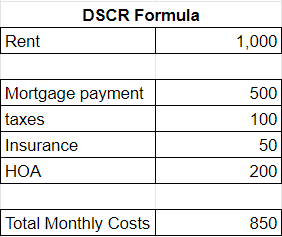## DSCR Calculation

Categories:

An important part of considering a DSCR loan is understanding the DSCR calculation. All lenders will look at this formula for DSCR loans.

Let’s go through and look at the numbers to find out if your property has enough cash flow for a DSCR loan.

## Income & Expenses

The number one thing DSCR lenders look at is income.

For this example, let’s say our rent is \$1,000 per month.

The next thing they look at is expenses.

They want to make sure your income more than covers your total costs. They’ll look at: mortgage payments, taxes, insurance, and HOA. Right now, they don’t look at property management costs, but that could change in the future.

Let’s fill out these numbers for our example property:So, the total expenses for this property are \$850. Right away, we can see that income more than covers expenses, and this property cash flows \$150/month.

## Applying the DSCR Formula

Then, the equation lenders will do to determine this cash flow will be:

Income  ÷  Expenses  =  Cash Flow Rate

Or, in this case:

1000  ÷  850  =  1.17+

Lenders are looking for a positive cash flow. They want properties with:

• Bare minimum: One-to-one. This means your rent at least covers your costs. (Example: Rent is \$1000 and your monthly expenses on the property is \$1000).
• Better: 1+
• Best: 1.25+